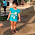## How To Work Out K-means Clustering Algorithm

k-means clustering aims to partition n observations into k clusters in which each observation belongs to the cluster with the nearest mean, serving as a prototype of the cluster.Distance measure here used is Euclidean, there are many other Distance measures used for k-means clustering.

Lets workout k-means using a simple example.
Problem 1:
Lets take a medicine example.

Here we have  4 medicine as our training data. each medicine has 2 coordinates, which represent the coordinates of the object.These 4 medicines belong to two cluster.We need to identify which medicine belongs to cluster 1 and cluster 2.

k-means clustering starts with a k value where k is user defined ie how many clusters . here we need to classify the medicines into 2 clusters so k = 2.

Next we need to identify initial centroids. lets selects the first 2 points as our initial centroids.

k = 2    Initial Centroids = (1,1) and (2,1)

## Iteration 1:

For each feature we need to calculate the distance to the cluster and find the minimum distance.

For Feature  (1,1)
C1 = (1,1)                                                                      C2 = (2,1)
= sqrt((1-1)^2  (1-1)^2)                                                     = sqrt((2-1)^2  (1-1)^2)
= 0                                                                                = 1

For Feature (2,1)
C1 = (1,1)                                                                      C2 = (2,1)
= sqrt((1-2)^2  (1-1)^2)                                                     = sqrt((2-2)^2  (1-1)^2)
= 1                                                                                = 0

For Feature  (4,3)
C1 = (1,1)                                                                      C2 = (2,1)
= sqrt((1-4)^2  (1-3)^2)                                                     = sqrt((2-4)^2  (1-3)^2)
= 3.6                                                                             = 2.82

For Feature (5,4)
C1 = (1,1)                                                                      C2 = (2,1)
= sqrt((1-5)^2  (1-4)^2)                                                     = sqrt((2-5)^2  (1-4)^2)
= 5                                                                                = 4.24

Next we need to identify the cluster having minimum distance for each feature.

C1 = (1,1)
C2 = (2,1),(4,3),(5,4)

Calculate the New Centroid till it converges.
New Centroids :
Continue the iteration.for all features with new centroids.

C1 remains the same.
For C2 the new centroid will be the average of the above listed points.
ie   (2+4+5/3 , 1+3+4/3)

C1 = (1,1)
C2 = (3.66,2.66)

## Iteration 2:

For each feature we need to calculate the distance to the cluster and find the minimum distance

Identify the cluster having minimum distance for each feature.

C1 = (1,1) , (2,1)
C2 = (4,3),(5,4)

New Centroids :
C1 = (1.5,1)
C2 = (4.5,3.5)
Check if the points converges.
How to check: Compare the old centroid with new one.If both are equal then k-means converges else continue the iteration.
Old Centroid :   (1,1) and (3.66,2.66)
New Centroid:  (1.5,1) and (4.5,3.5)

Old Centroid != New Centroid

Iteration continues.

## Iteration 3:

For each feature we need to calculate the distance to the cluster and find the minimum distance.

Identify the cluster having minimum distance for each feature.

C1 = (1,1) , (2,1)
C2 = (4,3),(5,4)

New Centroids :
C1 = (1.5,1)
C2 = (4.5,3.5)

Check if the points converges.

Old Centroid :   (1.5,1) and (4.5,3.5)
New Centroid:  (1.5,1) and (4.5,3.5)

Old Centroid = New Centroid

CONVERGES.
Therefore the algorithm stops.

Medicine A and B belongs to Cluster 1
Medicine C and D belongs to Cluster 2

1.hiii
good evening sreevani..kindly post the code in java for k-means algorithm in java

where can i run?? is it on mahout or normal hadoop/mapreduce

1.Siva you can find many source code related to kmeans over internet. Let me know if you need any help.

2.it not working
..i searched a lot
please send the code if u have
sivanagaraju46@gmail.com

2.Very much useful article. Kindly keep blogging

Java Training in Chennai

Java Online Training India